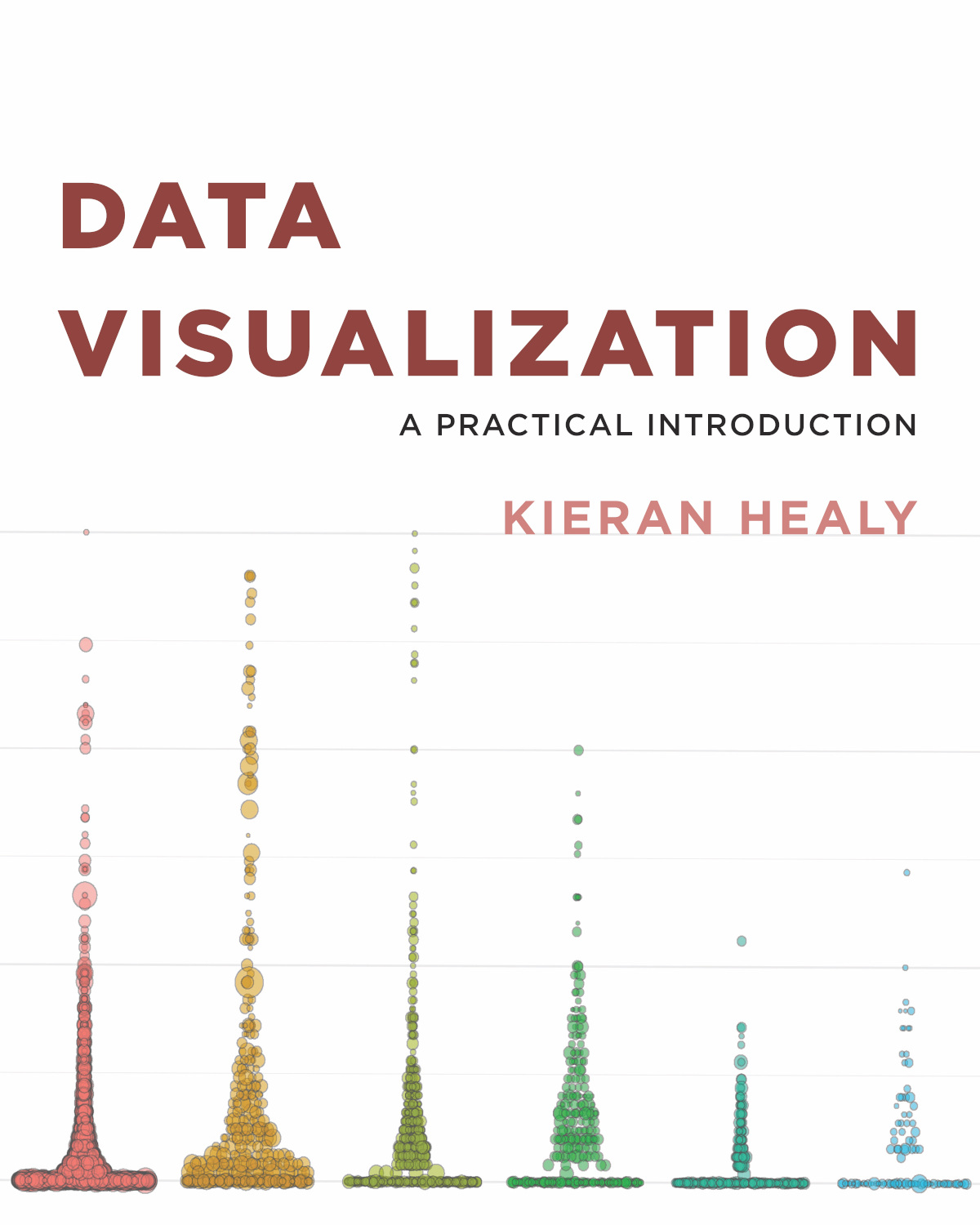# Chapter 3 Making Graphs

## 3.1 Class material

Note: most of this class talk in workshop style, so any recordings are short and the slides are brief

The live-coding demo used these files: class3a.R and class3b.R. These are based on Healy (2018) chapters 2 and 3 respectively.

## 3.2 Resources

This book is recommended for the course, and for this lecture in particular:Healy, K. (2018). Data visualization: a practical introduction. Princeton University Press.

## 3.3 Exercises

Review the introduction and Chapter 1 ‘Look At Data’ of Healy (2018)

Work through Chapter 2 ‘Get Started’ from 2.3 onwards (“Things to know about R”), we are ignoring the material on RMarkdown for now (we’ll get there, see here).

Work through Chapter 3 ‘Make a plot’

## 3.4 Checklist

Completed the exercises

Key concepts (chapter 2):

• variables and functions
• variables are typed (e.g. can be numeric or character)
• and how you detect what type a variable is
• indexing for variables and data frames

In the console, understood

• the shortcut keys for the assignment operator <-
• the use of tab for autocomplete
• the use up arrow to cycle through previous commands

Key concepts (chapter 3):

• Tidydata
• plots have data, aesthetic mappings (‘aes,’ ‘mappings’) and layers